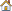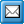﻿ SetCalculation
QPR Knowledge Base 2017.1

SetCalculationDeveloper's Guide > QPR API > The Object Model > QPR Metrics > SCModel Commands:

SetCalculationSetCalculationRevision history:

Introduced in QPR 7.3.1

Defines whether calculation is enabled for the model. If calculation is disabled, values can still be input, but formulas are not calculated automatically. Also updating of hierarchy tables is disabled in the case calculation is disabled. You need to use the Recalculate function to update the values acquired from formulas if calculation is disabled.

Synopsis:

SCModel.SetCalculation(viEnabled)

Parameters:

viEnabled: Defines whether calculation is enabled. Integer: 0 = disabled, 1 = enabled.

Required Rights:

Return Values:

Below are listed the return values that this function can return:

RV_APPLICATION_QUIT_CALLED

RV_NOT_CONNECTED_TO_SERVER

RV_NOT_AUTHENTICATED

RV_MODEL_NOT_OPEN

RV_SUCCESS

RV_NO_RIGHTS

RV_INVALID_PARAMETER

RV_CANNOT_LOCK_MODEL

RV_UNKNOWN_ERROR

Example Procedure:

iRet = SCModel.SetCalculation(1)